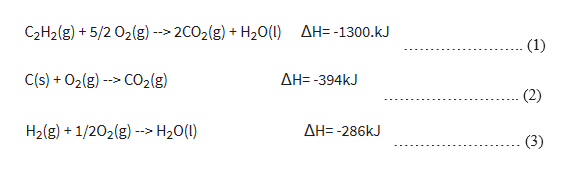# Given the following data:C2H2(g) + 5/2 O2(g) --> 2CO2(g) + H2O(l)      ΔH= -1300.kJC(s) + O2(g) --> CO2(g)                                   ΔH= -394kJH2(g) + 1/2O2(g) --> H2O(l)                                 ΔH= -286kJUse Hess’s Law to calculate ΔH for the reaction2C(s) + H2(g) --> C2H2(g)

Question

Given the following data:

C2H2(g) + 5/2 O2(g) --> 2CO2(g) + H2O(l)      ΔH= -1300.kJ

C(s) + O2(g) --> CO2(g)                                   ΔH= -394kJ

H2(g) + 1/2O2(g) --> H2O(l)                                 ΔH= -286kJ

Use Hess’s Law to calculate ΔH for the reaction

2C(s) + H2(g) --> C2H2(g)

check_circleExpert Solution
Step 1

The given equation are with their enthalpy of reaction arehelp_outlineImage TranscriptioncloseAH=-1300. kJ C2H2(g)+5/2 O2(g) --> 2CO2(g) +H20(1) (1) C(s) +O2(g)CO2(g) AH= -394KJ (2) H2(g) 1/202(g) > H20() AH= -286KJ (3) fullscreen
Step 2

The reaction to be obtained is

Step 3

To obtain the given reaction

Eq(4)= eq(...

### Want to see the full answer?

See Solution

#### Want to see this answer and more?

Solutions are written by subject experts who are available 24/7. Questions are typically answered within 1 hour*

See Solution
*Response times may vary by subject and question
Tagged in

### Chemical Thermodynamics